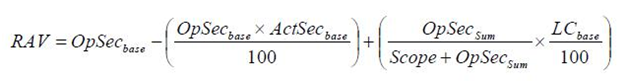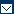## Calculating Risk Assessment ValuesTweet (function() { var po = document.createElement('script'); po.type = 'text/javascript'; po.async = true; po.src = 'https://apis.google.com/js/plusone.js'; var s = document.getElementsByTagName('script'); s.parentNode.insertBefore(po, s); })();

CEOs and project managers need to evaluate the risks associated with their decisions for best practices. There are three variables of best practices needed for calculating the Risk Assessment Value (RAV). These three variables are:

1.The Operational Security (OpSec): This is used to identify important information in order to determine whether a friendly approach can be used for preventing adverse effects. This best practice involves observation using an intelligence system to interpret the information to make it useful. Once the information is interpreted as useful, measures are taken to eliminate or minimize exploitation of the important information. This is an important risk management best practice. Operational Security has three variables:

i.      Visibility
ii.      Trust, and
iii.      Access

2.The Actual Security (ActSec): This best practice is simply described as the process of evaluating the actual risk associated with sensitive information. It can be existing risks of exploitation of the information. Calculating the ActSec is also an essential risk management best practice. There are five variables of Actual Security:

i.      Vulnerabilities
ii.      Weaknesses
iii.      Concerns
iv.      Exposures, and
v.      Anomalies

3.The Number of Loss Controls (LC): Risk management is all about implementing best practices using controls. There can be a number of controls managers implement in order to mitigate risks. Therefore, the number of loss controls is important in evaluating the Risk Assessment Values. There are ten variables of best practices associated with loss controls. These include:

i.            Authentication
ii.            Alarm
iii.            Confidentiality
iv.            Continuity
v.            Indemnification
vi.            Integrity
vii.            Privacy
viii.            Repudiation
ix.            Safety
x.            Usability

## The Equation for RAV

The equation for calculating RAV requires a base number to be assigned to each category of best practices. The base number may represent the protected scope (LCBase and OpSecBase), or it may represent the extent of risk caused (ActSecBase). Based on these evaluations, the following equation can be used to calculate the base numbers with best practices.

OpSecBase =             100 – OpSecSum

(Scope + OpSecSum)

LCBase =             Scope x LCSum x 0.1

Scope + OpSecSum

The LCSum must be multiplied by 0.1 in order to normalize the 10 categories into one single input. This is a very sensitive best practice for this calculation and must not be overlooked.

## Calculating ActSec

Calculating ActSec is done based on those values that distinguish between a verified problem and an identified problem for best practices. An identified problem is one that has been confirmed through an interview, an assumption or vulnerability detection. Verified problems are those that have been confirmed through manual processes by auditors. To calculate ActSecSum best practice variables the following values are to be used for the Actual Security base input in the RAV equation.

Calculating the ActSecSum is done by calculating it as the average value of each input and then multiplying it by the corresponding value. This is not a very difficult best practice. The equation is as follows:

ActSecBase =             ActSecSum

Scope

The RAV calculation corresponding to the above equation will then be:Distinguishing between the base number and the sum number is a very important best practice. Therefore, during calculations taking special note of these values a very crucial best practice for effective risk management.Contact Sitemap Links Copyright 2022 Best-Practice.com. All Rights Reserved.